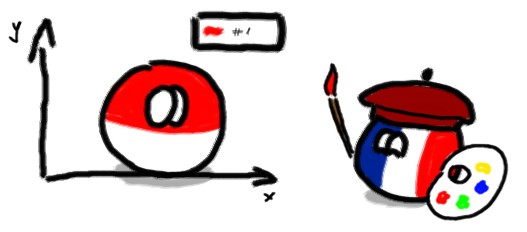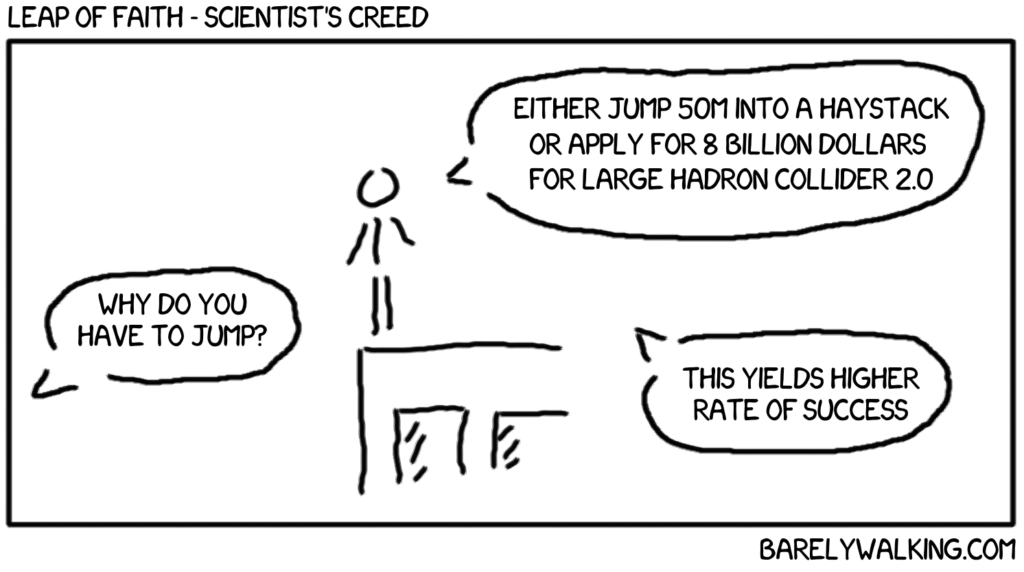Beautiful plots in Latexhttps://github.com/matlab2tikz/matlab2tikz – Matlab to TikZ (Latex graphics) conversion tool for plots
https://www.sharelatex.com/learn/TikZ_package – TikZ documentation – general graphics
https://www.sharelatex.com/learn/Pgfplots_package – Pgfplots documentation – plotting tool inside Latex

Modifications and examples below.

Plot names in Matlab – using the ‘Tag’ property

Did you know you can tag the plots in Matlab for easier searching? Because I fuckin’ didn’t.

Why so brutal? Well, after 6 years of writing your PhD in Matlab you CAN become furious when you realise instead of keeping the links to the currently opened plots in a global variables and different lists you could’ve been naming them all that time…

Tag the plot: description for searching

```aa = plot(2,2);
set(aa, 'Tag', 'foot trajectory' )
findobj('Tag','foot trajectory')
set( findobj('Tag','foot trajectory'), 'XData', [2 3], 'YData', '4 5')
```

… shit.

Matlab interface for Dynamixel motors and Bioloid robot

This is an old one, but is working quite nice and should be still useful for working with Dynamixel motor (AX-12+, AX-12A).
The goal was to have a nice toolbox used for direct control of Dynamixel motors using USB2Dynamixel or any other USB-Serial (half-duplex, ~1Mbps) adapter.

YARP, iCub + Matlab

All hail the mighty Matlab! … when it works

Ok, YARP and iCub-main are up and working, time for the jewel in the crown of every applied math-robotisct… robosicis… robotics mathematician: Matlab